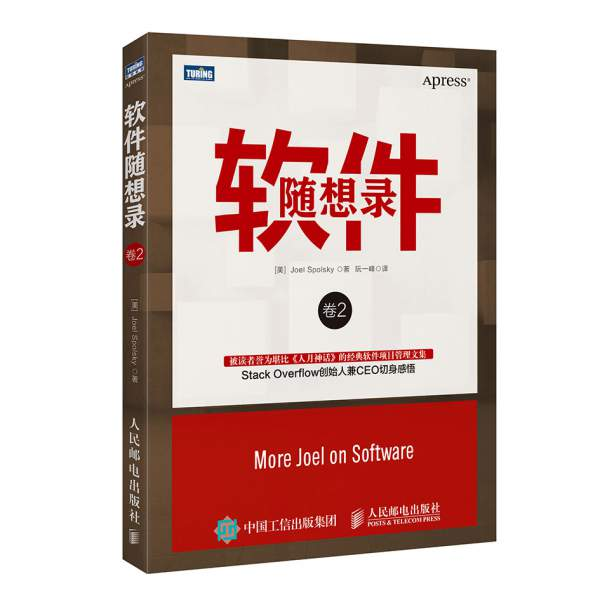# co 函数库的含义和用法========================================

## 一、什么是 co 函数库？

co 函数库是著名程序员 TJ Holowaychuk 于2013年6月发布的一个小工具，用于 Generator 函数的自动执行。``````
var gen = function* (){
console.log(f1.toString());
console.log(f2.toString());
};
``````

co 函数库可以让你不用编写 Generator 函数的执行器。

``````
var co = require('co');
co(gen);
``````

co 函数返回一个 Promise 对象，因此可以用 then 方法添加回调函数。

``````
co(gen).then(function (){
console.log('Generator 函数执行完成');
})
``````

## 二、 co 函数库的原理

（1）回调函数。将异步操作包装成 Thunk 函数，在回调函数里面交回执行权。

（2）Promise 对象。将异步操作包装成 Promise 对象，用 then 方法交回执行权。

co 函数库其实就是将两种自动执行器（Thunk 函数和 Promise 对象），包装成一个库。使用 co 的前提条件是，Generator 函数的 yield 命令后面，只能是 Thunk 函数或 Promise 对象。

## 三、基于 Promise 对象的自动执行

``````
var fs = require('fs');

return new Promise(function (resolve, reject){
if (error) reject(error);
resolve(data);
});
});
};

var gen = function* (){
console.log(f1.toString());
console.log(f2.toString());
};
``````

``````
var g = gen();

g.next().value.then(function(data){
g.next(data).value.then(function(data){
g.next(data);
});
})
``````

``````
function run(gen){
var g = gen();

function next(data){
var result = g.next(data);
if (result.done) return result.value;
result.value.then(function(data){
next(data);
});
}

next();
}

run(gen);
``````

## 四、co 函数库的源码

co 就是上面那个自动执行器的扩展，它的源码只有几十行，非常简单。

``````
function co(gen) {
var ctx = this;

return new Promise(function(resolve, reject) {
});
}
``````

``````
function co(gen) {
var ctx = this;

return new Promise(function(resolve, reject) {
if (typeof gen === 'function') gen = gen.call(ctx);
if (!gen || typeof gen.next !== 'function') return resolve(gen);
});
}
``````

``````
function co(gen) {
var ctx = this;

return new Promise(function(resolve, reject) {
if (typeof gen === 'function') gen = gen.call(ctx);
if (!gen || typeof gen.next !== 'function') return resolve(gen);

onFulfilled();
function onFulfilled(res) {
var ret;
try {
ret = gen.next(res);
} catch (e) {
return reject(e);
}
next(ret);
}
});
}
``````

``````
function next(ret) {
if (ret.done) return resolve(ret.value);
var value = toPromise.call(ctx, ret.value);
if (value && isPromise(value)) return value.then(onFulfilled, onRejected);
return onRejected(new TypeError('You may only yield a function, promise, generator, array, or object, '
+ 'but the following object was passed: "' + String(ret.value) + '"'));
}
});
``````

## 五、并发的异步操作

co 支持并发的异步操作，即允许某些操作同时进行，等到它们全部完成，才进行下一步。

``````
// 数组的写法
co(function* () {
var res = yield [
Promise.resolve(1),
Promise.resolve(2)
];
console.log(res);
}).catch(onerror);

// 对象的写法
co(function* () {
var res = yield {
1: Promise.resolve(1),
2: Promise.resolve(2),
};
console.log(res);
}).catch(onerror);
``````

（完）

## 留言（15条）

@秦先韩：

`引用Frank的发言：`

var g = gen();

g.next().value.then(function(data){
g.next(data).value.then(function(data){
g.next(data);
});
})

`引用Lluvio的发言：`

var g = gen();

g.next().value.then(function(data){
g.next(data).value.then(function(data){
g.next(data);
});
})

`引用hacke2的发言：`

`引用jane的发言：`

«-必填

«-必填，不公开

«-我信任你，不会填写广告链接